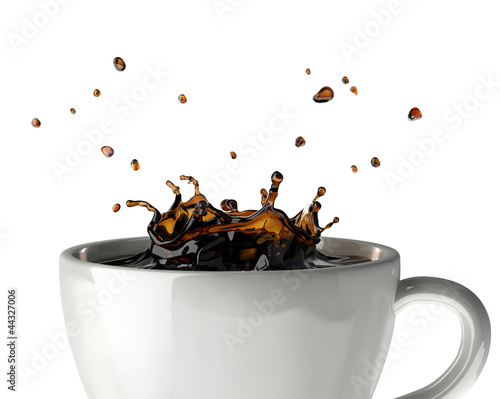# sandbox/Antoonvh/splash.cOne may wounder where the fluid from the impacting droplet goes during a splash. Image via adobe stock.

# A splash

A droplet impacts on a pool and creates a splash. We are curious to see where the fluid of the droplet ends up after the flow has settled a bit. For that purpose, we add particle tracers to the fluid in the droplet.

Drop-fluid tracers and the interface

Furthermore, tracer particles just outside the drop are added to see how their paths divergce from their initially-nearby neighbors at the other side of the interface.

All tracers and the interface

#include "navier-stokes/centered.h"
#include "two-phase.h"
#include "tension.h"
#include "reduced.h"
#include "view.h"
#include "vof-tracer-particles.h"
#include "scatter2.h"

Particles Pin, Pout;
int maxlevel = 9;

The system parameters are chosen adhoc to produce a splashing scenario.

int main() {
L0 = 20;
X0 = -L0/2;
Y0 = -2;
f.sigma = 10.;
mu1 = .5;
mu2 = .5;
rho1 = 50.;
rho2 = 1.;
G.y = -5.;
init_grid (64);
run();
}

event init(t=0) {
refine (sq(x) + sq(y) < 1.1 && level < maxlevel);
fraction (f, 1 - sq(x) - sq (y - 5)); // The drop
scalar m[];
fraction (m, -y); // and the pool
foreach()
f[] += m[];
DT = 0.05;

We add 2 times 99999 tracers: First, they are distributed rondomly within the circular drop (Pin). Second, a ring surrounding the drop ais “filled” with particles (Pout).

  int Pnr = 99999;
Pin = new_vof_tracer_particles (Pnr, 1); //Assign phase f[] = 1
place_in_circle (Pin, (struct Init_P){Pnr, 0, 5, 1});
particle_boundary (Pin);

Pout = new_vof_tracer_particles (Pnr, 0); //assign phase f[] != 1
foreach_particle_in(Pout) {
double R = 1.1 + 0.1*noise();
double angle = pi*noise();
p().x = R*sin(angle);
p().y = R*cos(angle) + 5;
}
particle_boundary (Pout);
}

adapt_wavelet ((scalar*){f,u}, (double[]){0.001, 0.1, 0.1}, maxlevel);

event bviewer (t += 0.05) {
translate (z = 0.05)
draw_vof ("f", lw = 3);
scatter (Pin, s = 5, pc = {0.6, 0.4, 0.6});
scatter (Pout, s = 5, pc = {0.6, 0.6, 0.4});
save ("mov_both.mp4");

clear ();
translate (z = 0.05)
draw_vof ("f", lw = 3);
scatter (Pin, s = 5, pc = {0.6, 0.4, 0.6});
save ("mov.mp4");
}

event stop (t = 30) {
return 1;
}